Mechanics Index
Simple Struts

Introduction..... Symbols..... Eulers Theory..... Rankine - Gordon Criteria..... Perry Robertson formula .....

Introduction

A long structural member subject to a compressive load is called a strut.   Struts with large cross sections compared with the length generally fail under compressive stress and the conventional failure criteria apply.  When the cross section area is not large compared to the length i.e the member is slender, then the member will generally fail by buckling well before the compressive yield strength is reached.

The notes below relate to uniform straight members made from homogeneous engineering materials used within the elastic operating range. It is assumed that an end load is applied along the centroid of the ends.  The strut will remain straight until the end load reaches a critical value and buckling will be initiated.  Any increase in load will result in a catastrophic collapse and a reduction in load will allow the strut to straighten.   The value of the critical load depends upon the slenderness ratio and the end fixing conditions.  The slenderness ratio (λ )is defined as the effective length =Le / the least radius of gyration = k of the section  The principal end fixing conditions are listed below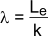The principal end fixing conditions are listed below

 Pinned (hinged) at both ends Fixed (built-in) at both ends Fixed at one end and free at the other Fixed at one end and pinned at the other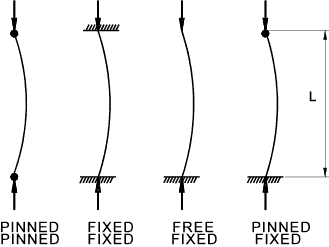Symbols
 A = Area (m2) k = radius of gyration of section (m) W = End force on strut. (N) We = Euler end force on strut. (N) WR = Rankine end force on strut. (N) L = Length of strut (m ) Le = effective length of strut (m) x = position along strut y = strut deflection at x R= Radius of bend (m) Pc= Allowable stress (N/m2 ) M = moment (Nm) I = Moment of inertia (m4) σ e = Euler stress (N/m2) σc = compressive failure stress (N/m2) σR = compressive failure stress (N/m2) λ = slenderness ratio E = modulus of elasticity (Young's Modulus) (N/m2) Wc = Compressive failure Load We = Euler Load

Eulers Theory

The simple analysis below is based on the pinned-pinned arrangement.  The other arrangements are derived from this by replacing the length L by the effective length Le.

For the pinned-pinned case the effective length Le = L.
For the Fixed -Fixed case the effective length Le = L/2.
For the Fixed-Free case the effective length Le = L x 2.
For the Fixed-Pinned case the effective length Le approx. L x 0,7.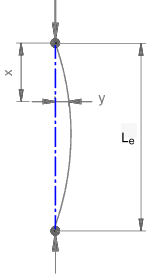Curvature

Quick derivation for curvature (1/R)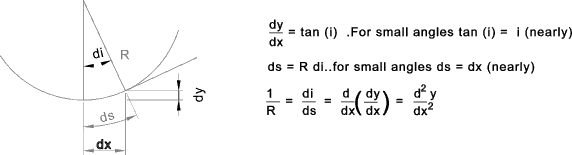Beam equation

Note: The derivation below is based on a strut with pinned ends. A similar method can be used to arrive at the Euler loads for other end arrangements which will confirm the basis for the factors in arriving at the equivalent length b.

M / I = σ / y = E / R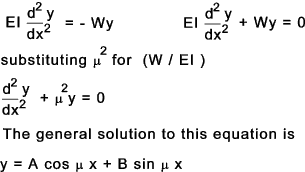When x = 0 y = 0 and therefore A cos μ.0 + B sin μ.0 = A = 0 therefore A = 0
When x = b , y = 0 and so B sin μb = 0.
B cannot be 0 because there would be no deflection and no buckling which is contrary to experience.
Hence sin μ Le = 0. therefore μ Le = 0, π, 2π, 3 π etc

(W/EI) Le 2 = π 2, 4.π 2, 9.π 2 etc
therefore
W = π 2 E.I / Le2 , π 2 E.I / (Le / 2) 2, π 2 E.I / (Le / 3) 2
The lowest value of W results from π 2 E.I / Le2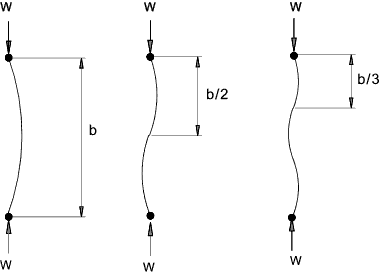The lowest value of W resulting from this procedure is called the Euler load (We ) and failure of long slender beams due to buckling results from this much earlier than failure due pure compression.
As the moment of inertia I = A.k 2 and the end force W = σ A. The formula can be rewritten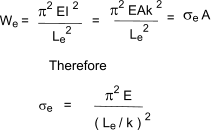Important Note: The value of I and the equivalent value of k are assumed to be the minimum values for the section under consideration

Validity of Eulers theory

This theory takes no account of the compressive stress.   For a metal with a compressive strength of less than 300 N/mm2 and a Young's Modulus of about 200 kN/mm2.    The strut will tend to fail in compression if the slenderness ratio (Le/ k) is less than 80.    Therefore for steel Eulers equation is not reliable for slenderness ratios less than 80 and really should not be used for slenderness ratios less than 120.

Rankine - Gordon Criteria

This criteria is based on experimental results.

This criteria suggests that the strut will fail at a load given by.

1 / W R = 1 / Wc + 1 / We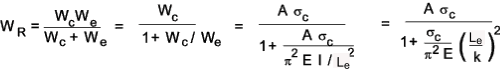Substituting c = σ c / ( π 2 E) - A constant for each material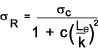This design criteria provides more accurate buckling loads than the euler theory especially at lower slenderness ratios.  At higher slenderness ratios the two methods yield similar results. The experimental values for c are not in direct agreement with the theoretical values. BS 449-2:1969 includes tables for the safe working stresses for all slenderness ratios and a range of steel specifications.

Table showing approximate values of c
 Material c Mild Steel 1/7500 Wrought Iron 1/9000 Cast Iron 1/1600 Wood 1/3000

Perry Robertson formula (BS 449-2 )

Important ..The notes and equation and table below is provided for general guidance. For detail structural design it is important to refer to the identified standards.   The information below is only a trivial relative to the level of detail provided in the standard.

The equation below is used as the basis for the allowable design stresses as provided in the relevant tables in BS 449 and is considered the most reliable of the methods available for buckling loads for long slender struts..The equation below is similar to that provided in appendix B of BS 449 part 2 :1969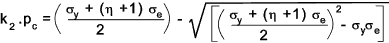pc = Permissible average compressive stress
k2 = Load coefficient ( normally 1,7)
σ y = Yield stress N/m2
σ y = Euler stress N/m2
η = 0,3 ( Le / 100k )2

Table of Allowable stress values pc for structural steels

The table is based on table 17 in BS 449 and relates to BS 4360 steels. Which is superseded by BS EN 10025 the grades of which are identified ().

 Grade 43 (S275) Grade 50 (S355) Grade 55 (S420) Grade 43 (S275) Grade 50 (S355) Grade 55 (S420) Le/ k MPa Le/ k MPa 0 170 215 265 95 89 100 108 5 167 212 262 100 82 92 99 10 165 210 258 110 71 78 83 15 162 207 255 120 62 67 71 20 159 205 252 130 54 58 61 25 157 202 248 140 47 50 53 30 154 200 245 150 41 44 46 35 152 197 241 160 37 39 41 40 150 193 236 170 33 35 36 45 147 189 230 180 29 31 32 50 144 184 222 190 26 28 29 55 139 177 212 200 24 25 26 60 135 169 200 210 22 23 24 65 129 160 186 220 20 21 22 70 123 150 172 230 18 19 20 75 116 140 157 240 17 18 18 80 109 129 143 250 16 16 17 85 102 119 130 300 11 11 12 90 95 109 118 350 8 8 9
 Links to Struts DANotes - Buckling ..Relevent detail reference document Buckling of Slender Struts ..Faculty of Technology Plymouth -Lecture Notes MITcalc ..A vey useful Excel based software suite which includes and excellent section on buckling

Mechanics Index